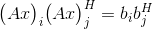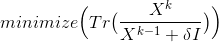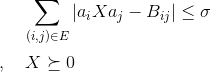# Convexity, Semidefinite Programming and rank-minimization

Hi there!
I’m an electrical engineering student trying my hands on optimisation, and would like to solve a system with quadratic combinations of data contained in a vector b, as seen below.I really tried finding my answers in the book of Boyd and Vandenberghe, and other littereture on the topic, but I am finding it hard to sort out the relevant information in all the new stuff I am reading. Therefore I thought I’d try my luck here.

For solving this problem i i found a rank minimization technique in here:
In "M.Fazel, H.Hindi, and S.Boyd,“Rank minimization and applications in system theory,” presented at the Amer. Contr. Conf. Boston, MA, USA, Jun. 2004. , and uses this to encourage low-rank solution, since I have relaxed my problem with a semidefinite relaxation.

My optimization problem then looks as follows:subject toThe idea is to be able to choose specific quadratic combinations of the vector b with a “selector matrix” E.
Se code below (implemented in MATLAB)

``````    cvx_begin quiet
variable X(sysdata.N,sysdata.N) hermitian semidefinite
minimize( trace((X_last+sysdata.delta*I)^(-1)*X) )
subject to
for i = 1:sysdata.M
for j = 1:sysdata.M
if sysdata.E(i,j) == 1
Qij = A(j,:)'*A(i,:);
abs(trace(Qij*X)-B(i,j)) <= sig;
end
end
end
X == hermitian_semidefinite(sysdata.N)
cvx_end
``````

My questions:

Program is running rather slowly, is there any way to speed it up?
How is my problem convex or what ensures the convexity?

Rasmus

What solver are you using? Try Mosek if it is available to you and you have CVX Professional or Academic.

Remove the quiet option until you have the program working well. By doing so, you will see the solver and CVX output. Perhaps some readers can offer guidance if they see the solver output.

You haven’t shown it, but I presume you will CVX inside a loop, and will have to specify an initial value of X_last prior to first use. If so, it looks like you are performing successive convex programming, which lets you try to find a locally optimal solution to a non-convex problem (which you would have if the objective function had X in place of X_last). The performance of such an algorithm can be strongly influenced by the quality of the initial value for X_last… What are the numerical values of sysdata.N and sysdata…M, i.e., how large is your problem?

I don;t knwo whether it makes any differ3encde in your case, but it might be better to use equation solve rather than multiplying by matrix inverse in the objective function. The argument of trace in the objective function seems ambiguous as to the exact matrix calculation you want, and therefore whether you should be using \ or / for the equation solve.However, your code is unambiguous in the sense that it specifies a specific calculation to be performed, but that might not be the one you really (should) want. But it is your problem, so I will let you figure that out. You can;t be as cavalier with matrix “division” as you can with scalar division. Order matters.

Dear Mark.

You’re right, I forgot to include my loop arround the code (Note that I is the identity matrix)
X_last = I;
for k = 1:sysdata.iter_rankmin
cvx_begin quiet
variable X(sysdata.N,sysdata.N) hermitian semidefinite
minimize( trace((X_last+sysdata.delta*I)^(-1)*X) )
subject to
for i = 1:sysdata.M
for j = 1:sysdata.M
if sysdata.E(i,j) == 1
Qij = A(j,:)'A(i,:);
abs(trace(Qij
X)-B(i,j)) <= sysdata.sig;
end
end
end
X == hermitian_semidefinite(sysdata.N)
cvx_end
X_last = X;
end

The size of my program is at the moment quite small.
sysdata.M = 10 and sysdata.N = 5
But I would like to be able to do problems with a size of
sysdata.M = 10 and sysdata.N = 1000

Regarding your comment about using equation solve. Is it correctly understood that the motivation is to be sure to get the results that I want, and not necessarily speeding up the calculations?

Equation solve is numerically better behaved than use of inverse,

M,y pother point is that the algebraic formula shown in you r first post for the argument of the trace is ambiguous. Unlike with scalars, Order matters when dealing with matrix “division” (inversion) - so I don;t know what you really want. it is your model so that is your responsibility.